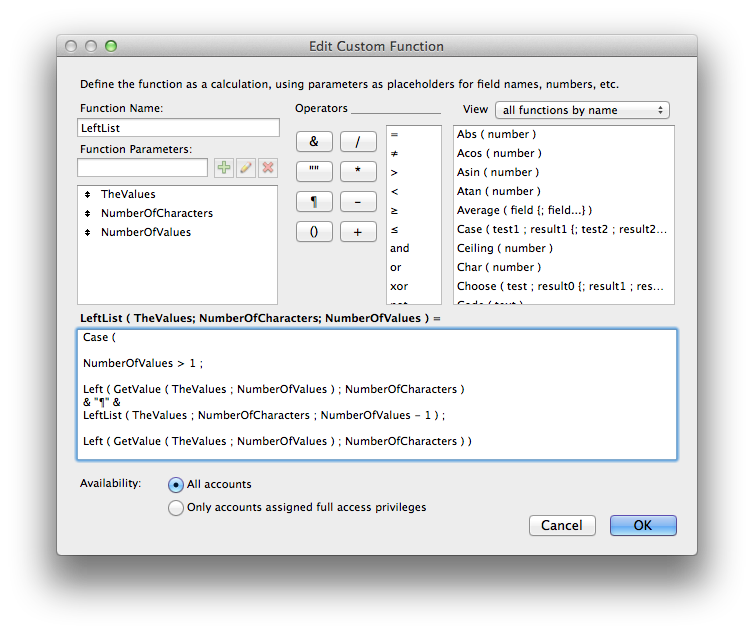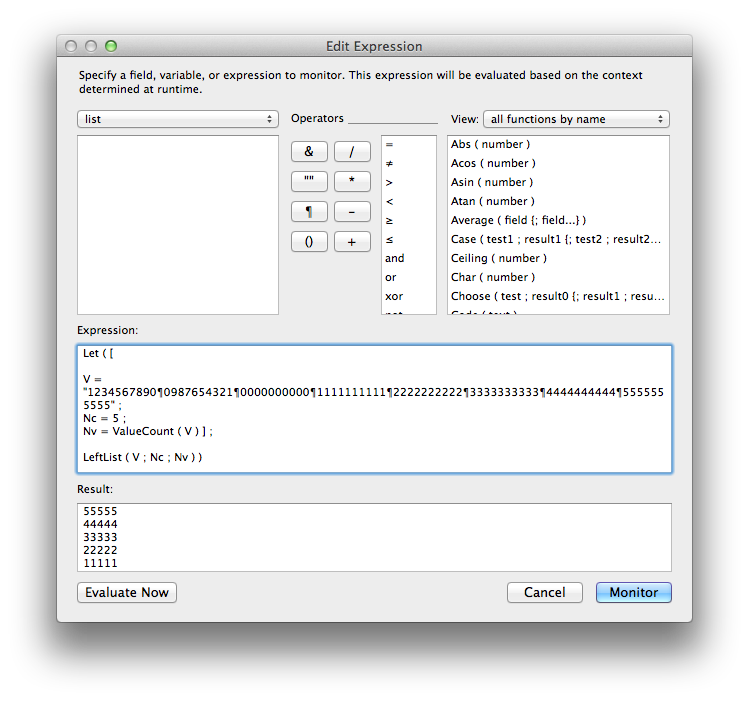# FileMaker ForumSo, you want to alter each value in a return delimited list? The easiest way to accomplish that would be to use a custom function to alter each value one at a time. The custom function would need to isolate each value and alter it according to your needs (get the left 10 characters). Here's what I came up with:CUSTOM FUNCTION: LeftList ( TheValues ; NumberOfCharacters ; NumberOfValues ) Case ( NumberOfValues > 1 ; Left ( GetValue ( TheValues ; NumberOfValues ) ; NumberOfCharacters ) & "¶" & LeftList ( TheValues ; NumberOfCharacters ; NumberOfValues - 1 ) ; Left ( GetValue ( TheValues ; NumberOfValues ) ; NumberOfCharacters ) )EXAMPLE USE: Let ( [ V = "1234567890¶0987654321¶0000000000¶1111111111¶2222222222¶3333333333¶4444444444¶5555555555" ; Nc = 5 ; Nv = ValueCount ( V ) ] ; LeftList ( V ; Nc ; Nv ) ) OUTPUT: 55555 44444 33333 22222 11111 00000 09876 12345Kevin Kurpe